# Gallery: Moving axes

## 1) Moving the y axis

In this example we plot up a Gaussian distribution and move the Y axis so that it is at the peak of the distribution.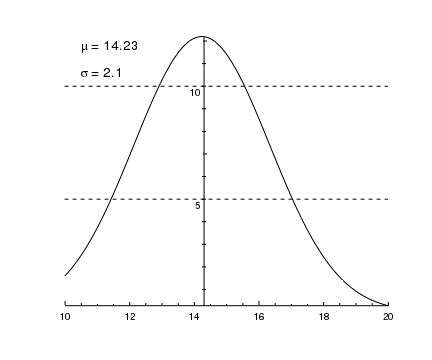Version: Postscript; PDF
# Create a Gaussian
x = np.linspace(10, 20, 100)
xmean = 14.23
xsigma = 2.1
y = 12.2 * np.exp(-0.5 * (x-xmean) * (x-xmean) / (xsigma * xsigma))

# Plot the data

# Move the Y axis and make sure the tick marks appear on
# both sides of the axis
cid = ChipsId()
cid.axis = 'ay1'
cid.coord_sys = DATA
move_axis(cid, xmean, 0)
set_yaxis(["tickstyle","centered","minortick.length",4])

# Hide the plot borders and remove the axis padding
set_plot(["style","open"])

# Change the mode used to label the Y axis, add on the
# major grid lines, then move the tick labels vertically
# down so that they do not overlap the grid lines
set_yaxis(["majortick.interval",5,"majorgrid.visible",True])
set_yaxis(["ticklabel.valign",1])

# Add labels to the top-left of the plot
lopts = ['coordsys', PLOT_NORM, 'size', 18]
add_label(0.05, 0.95, r"\mu = {}".format(xmean), lopts)
add_label(0.05, 0.85, r"\sigma = {}".format(xsigma), lopts)


In many of the examples in this section, we can call move_axis without a ChipsId argument, but here we have to since we need to specify both the axis to move (ay1; the Y axis) and the name of the coordinate system (DATA, since the default system for move_axis is PLOT_NORM).

After moving the axis and adjusting it so that the tick marks lie on both sides of the axis, as well as doubling the length used for these marks, we change the mode used to calculate where to place the major tick marks. Setting the majortick.interval attribute of the Y axis also changes its majortick.mode attribute to interval. This means that we want a major tick mark every 5 (the default setting uses a major tick mark every 2 for this example). See the examples in the Axis styles and grids section for more information on changing the majortick mode.

Setting the majorgrid.visible attribute to True, horizontal grid lines are displayed at every major tick position. As these lines overlap the tick labels on the Y axis, we change the vertical alignment of the labels (with respect to the major tick mark) by setting ticklabel.valign to 1.

The plot is finished off by placing two labels in the top-left corner using plot-normalized coordinates.

## 2) Moving the axes so they go through (0,0)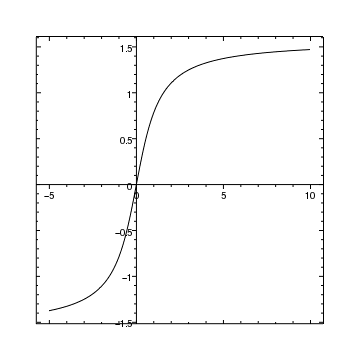Version: Postscript; PDF
add_curve("atan.fits[cols X,Y]",["symbol.style","none"])

set_plot(["leftmargin",0.1,"bottommargin",0.1])

# Move both axes so that they are at x=0 and y=0
# in data coordinates
cid = ChipsId()
cid.coord_sys = DATA
move_axis(cid,0,0)

# Ensure the tick marks and labels are on the left of, or
# below, the axes
set_axis(["majortick.style","outside","minortick.style","outside"])


When the axes were created for this plot they were placed using the plot-normalized coordinate system (e.g. the X axis was placed at yplot normalized = 0). This means that, unless explicitly specified, the move_axis call will assume the coordinates it is given are also in the plot-normalized coordinate system. Since we want to move the axes to (0,0) in the data coordinate system, we have to send in a ChipsId structure with the coord_sys field set to DATA.

Whilst the axes move, the borders - in this case "bx1" and "by1" - remain, which is why there are tick marks around all four edges of the plot. The other examples in this section show how the border properties can be changed (Moving the axes and using a boxed border) or hidden completely (Moving the axes with an open border), as well as how to tweak the axis properties to clean up the display (Adjusting axis labels).

In this example we take the previous plot and use the set_arbitrary_tick_positions routine to avoid the label/axis overlap at the origin.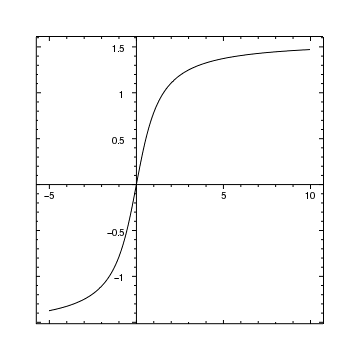Version: Postscript; PDF
add_curve("atan.fits[cols X,Y]",["symbol.style","none"])

set_plot(["leftmargin",0.1,"bottommargin",0.1])

cid = ChipsId()
cid.coord_sys = DATA
move_axis(cid,0,0)

set_axis(["majortick.style","outside","minortick.style","outside"])

# Tweak the Y axis so that the y=-0.5 label is not
# obscured by the curve
set_yaxis(["ticklabel.offset",15])

# Remove those Y axis labels which overlap other plot
# elements.
ypos = np.arange(-2, 2.1, 0.5)
ylbl = ["", "", "-1", "-0.5", "", "0.5", "1", "1.5", ""]
set_arbitrary_tick_positions("ay1",ypos,ylbl)

# Repeat for the X axis
xpos = np.arange(-10, 16, 5)
xlbl = ["", "-5", "", "5", "10", ""]
set_arbitrary_tick_positions("ax1",xpos,xlbl)


The three changes to the previous plot are:

1. the ticklabel.offset attribute of the Y axis is increased so that the y=-1.5 label does not overlap the curve;

2. the Y axis labels are adjusted to hide the -1.5 and 0 labels;

3. and the X axis labels are adjusted to hide the 0 label.

As the set_arbitrary_tick_positions command only draws minor tick marks between the locations given to it (i.e. the second argument), we have included values not shown on the plot; so for the Y axis the positions include -2 and 2 even though the plot range is only -1.5 to 1.5. Since these values are not going to be displayed, they are set to the empty string (""). The NumPy documentation for arange explains why the upper limit used (2.1 and 16) are larger than the maximum value required.

## 4) Moving the axes and using a boxed border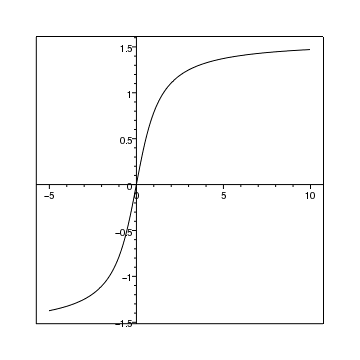Version: Postscript; PDF
add_plot(["style","boxed"])

cid = ChipsId()
cid.coord_sys = DATA
move_axis(cid,0,0)

set_axis(["majortick.style","outside","minortick.style","outside"])
set_plot(["leftmargin",0.1,"bottommargin",0.1])


## 5) Moving the axes with an open border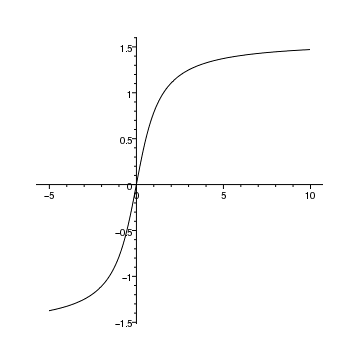Version: Postscript; PDF
plt = ChipsPlot()
plt.style = "open"
crv = ChipsCurve()
crv.symbol.style = "none"

cid = ChipsId()
cid.coord_sys = DATA
move_axis(cid,0,0)

set_axis(["majortick.style","outside","minortick.style","outside"])
set_plot(["leftmargin",0.1,"bottommargin",0.1])


## 6) Move axes away from the plot

In this plot we move the axes away from the plot area slightly, which can be useful in identifying objects at the edges of the plots.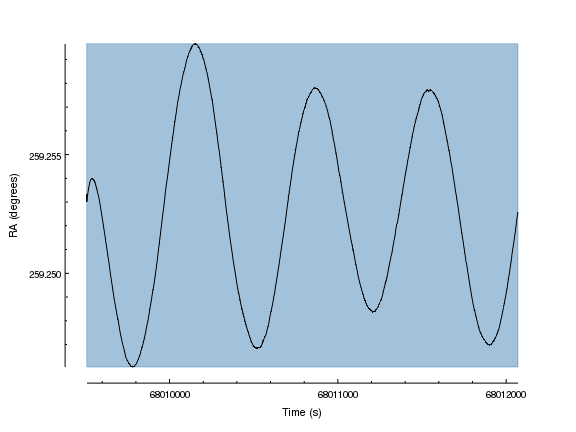Version: Postscript; PDF
add_window(8,6,"inches")

set_plot(["style","open"])

set_xaxis(["majortick.interval",1e3,"tickformat","%.0f"])
set_yaxis(["majortick.interval",5e-3,"tickformat","%.3f"])
set_yaxis(["minortick.count",4])

set_plot_xlabel("Time (s)")
set_plot_ylabel("RA (degrees)")
set_yaxis(["offset.perpendicular",60])

# Move the axes away from the plot; the coordinates are in
# the plot-normalized coordinate system and the final 1 indicates
# these are relative values for the move.
move_axis("ay1",-0.05,0,1)
move_axis("ax1",0,-0.05,1)

# Add a region covering the plot area, making sure it is
# behind the curve
xr = [0, 1, 1, 0]
yr = [0, 0, 1, 1]


After creating the plot, we use the move_axis call to move the Y and then X axis slightly away from the plot area.

The axes can only be moved vertically (for X) and horizontally (for Y), so the "other" coordinate in the move_axis call should be 0. The default coordinate system for the move is plot-normalized, so that 0 indicates the bottom, or left, edge of the plot and 1 is the top, or right, edge. See some of the early examples in this section for examples using the data coordinate system to move axes.

The last argument, which is optional, indicates whether the values are absolute (the default) or relative; here we give relative coordinates (i.e. the distance to move) so give a 1. However, since the axis was originally positioned at a coordinate of 0, then in this case the result is the same as if were specifying the absolute coordinate (e.g. see the calls in later examples).

A region is used to indicate the plot area using the approach explained in the adding a background to a plot example. Although the region is partially opaque, on screen and in the PDF and bitmap outputs, it would obscure the PS output, so it is moved behind the curve by setting its depth to 50.

## 7) Move axes away from the plot

This is very similar to the previous example; the main difference here is that the tick marks are moved outward and the limits changed to ensure the axes start and end at a major tick position.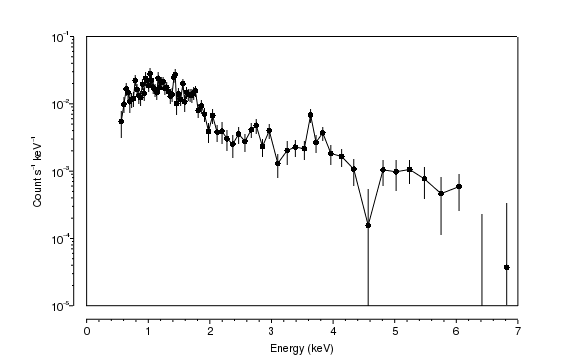Version: Postscript; PDF
add_window(8,5,"inches")

set_curve(["symbol.style","circle","symbol.size",3])
log_scale(Y_AXIS)

set_plot_xlabel("Energy (keV)")
set_plot_ylabel("Count s^{-1} keV^{-1}")

# Here we move the axes to an asbolute position.
move_axis("ay1",-0.03,0)
move_axis("ax1",0,-0.05)

# Change the plot style so that it draws a box rather than
# the border axes
set_plot(["style","boxed"])

# Adjust the tick marks so that they point outside rather
# than inside
set_axis(["tickstyle","outside"])

# Adjust the limits so that they start/end on a major tick mark.
# An alternate approach would be to use
#   set_axis(['majortick.mode', 'nice'])
limits(X_AXIS,0,7)
limits(Y_AXIS,1e-5,0.1)

# Adjust the Y axis to use scientific notation for the tick labels,
# and increase their font size.
set_yaxis(["tickformat","%0.0z"])
set_axis(["ticklabel.size",14])


In this example we miss off the optional last argument to move_axis, so that the coordinates are taken to be absolute (for axes this only matters if you wish to adjust an axis that has already been moved, since the initial position is at 0, so the absolute and relative coordinates are the same in this case).

Once the axes have been moved, the tickstyle attribute of both axes is changed so that the tick marks point away from - rather than towards - the plot, and the limits are set so that the axes start and end at a major tick mark. As noted in the code, we could have said

set_axis(['majortick.mode', 'nice'])

to get the same result for the X axis, which uses a linear scale, but the Y axis in this case would still need adjusting, since the "nice" mode does not work so well for logarithmically-scaled axes. The Axis styles and grids section of the gallery contains examples highlighting the various modes available for choosing tick marks.

The plot is finished off with minor adjustments to change the format of the Y axis and the font size used for the numeric labels on both axes.

## 8) Moving axes away from an image

Here we move the axes around a plot containing an image; in this case the Galex image used in the Combining images and annotations section of the 'Displaying image data' thread.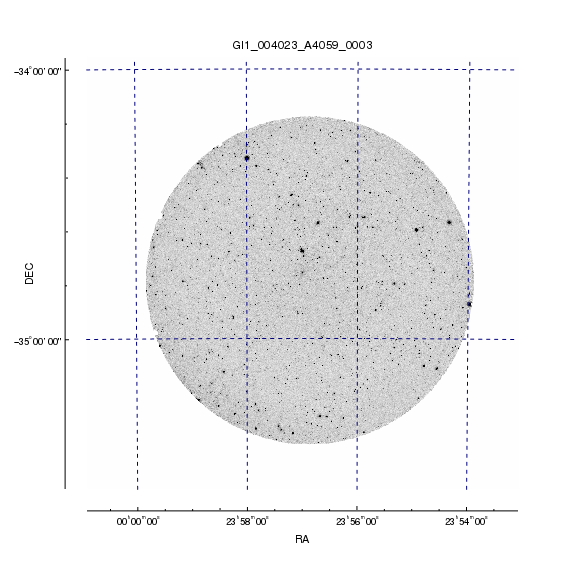Version: Postscript; PDF
add_window(8,8,"inches")
make_figure("a4059_galex.fits[bin #1=::4,#2=::4]","image",["depth",50])
set_image(["threshold",[0, 0.2],"invert_colormap",True])

# Move the axes
move_axis("ax1",0,-0.05)
move_axis("ay1",-0.05,0)

# Hide the plot edges
set_plot(["style","open"])

# Draw the major grid lines, ensuring they can be seen on
# screen and in the hardcopy output
set_axis(["majorgrid.visible",True,"majorgrid.color","navy"])

set_xaxis(["tickformat","%ra"])
set_yaxis(["tickformat","%dec"])


Since the Galex image is large - almost 4000 pixels square, as shown below - we take advantage of the CIAO binning support in the Data Model to re-bin the image by a factor of 4 on each axis.

unix% dmlist a4059_galex.fits blocks

--------------------------------------------------------------------------------
Dataset: a4059_galex.fits
--------------------------------------------------------------------------------

Block Name                          Type         Dimensions
--------------------------------------------------------------------------------
Block    1: PRIMARY                        Image      Real4(3840x3840)
unix% dmlist "a4059_galex.fits[bin #1=::4,#2=::4]" blocks

--------------------------------------------------------------------------------
Dataset: a4059_galex.fits
--------------------------------------------------------------------------------

Block Name                          Type         Dimensions
--------------------------------------------------------------------------------
Block    1: PRIMARY_IMAGE                  Image      Real4(960x960)


After displaying the image and moving the axes, we turn on the major grid lines (this is the reason for making sure the image was created with a depth of 50, so that it would not obscure the grid lines). These grid lines are restricted to the plot area, even though the axes themselves have been moved outside this region.